Miscellaneous

Chapter 5 Class 11 Complex Numbers (Term 1)
Serial order wise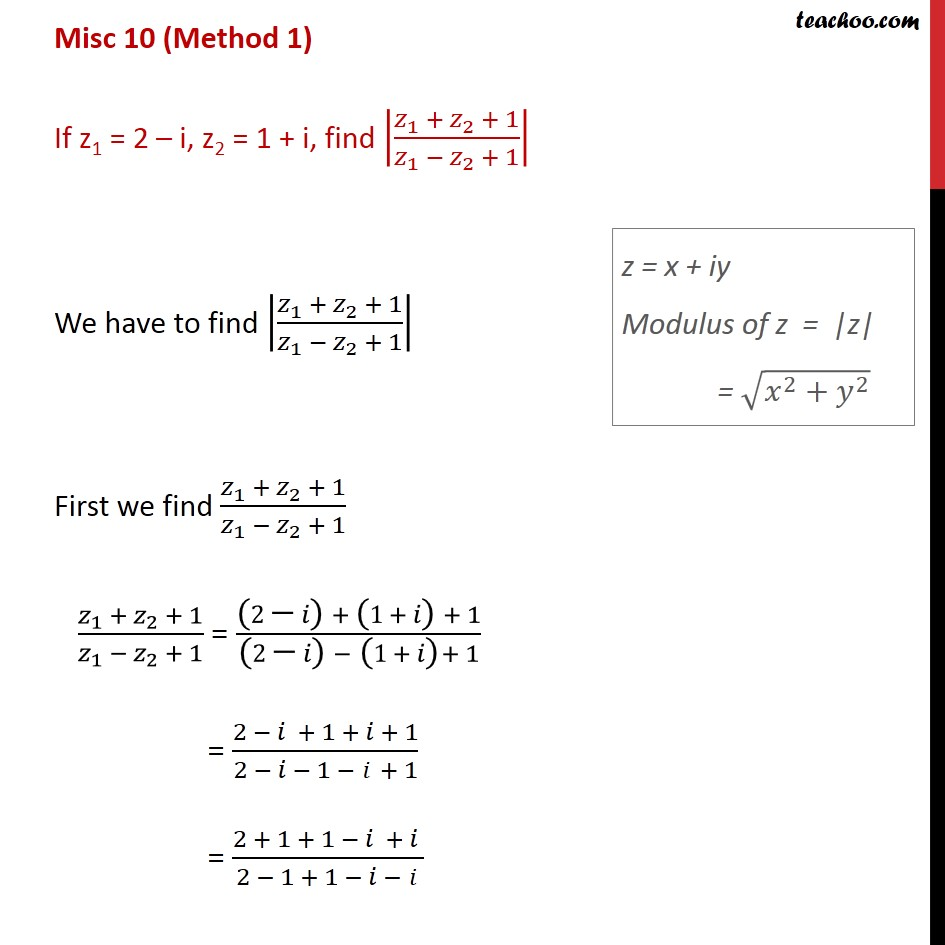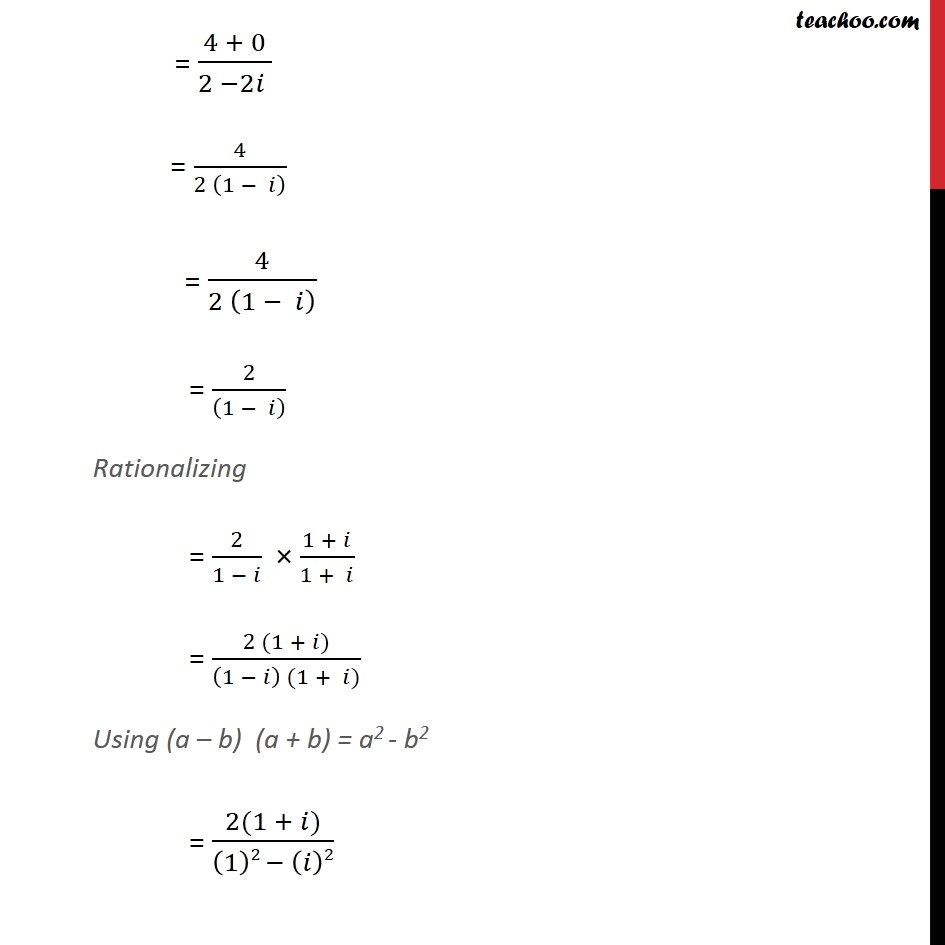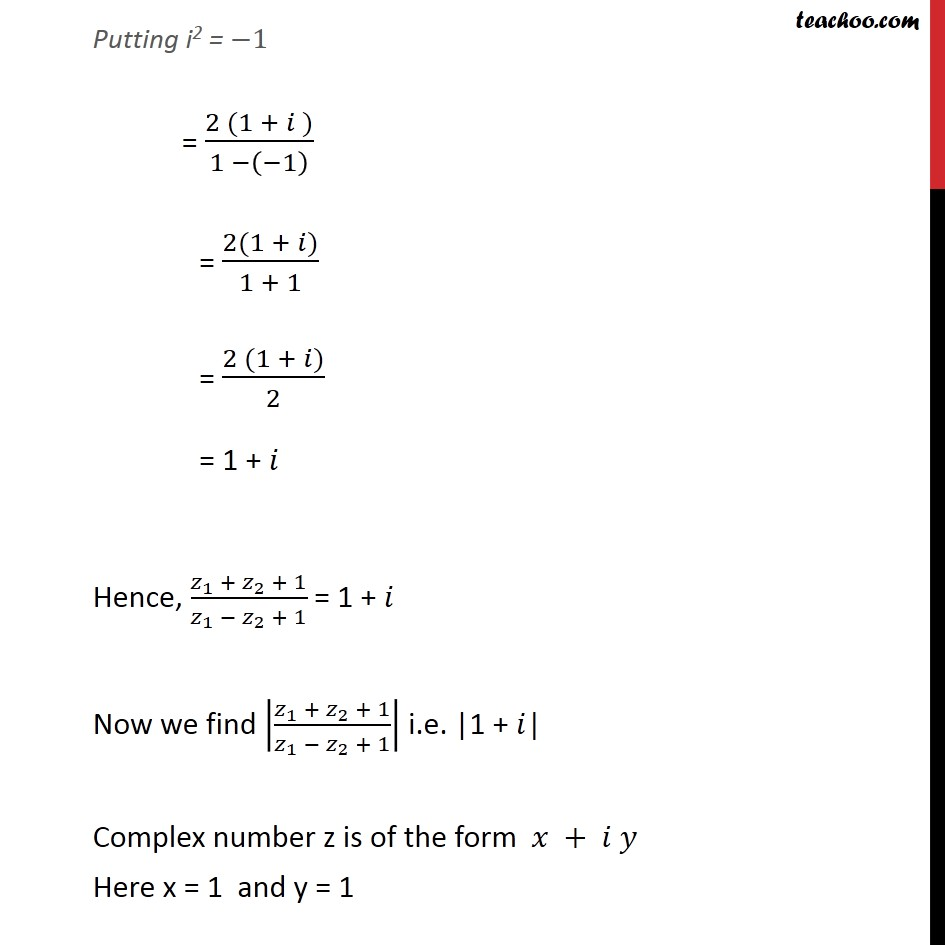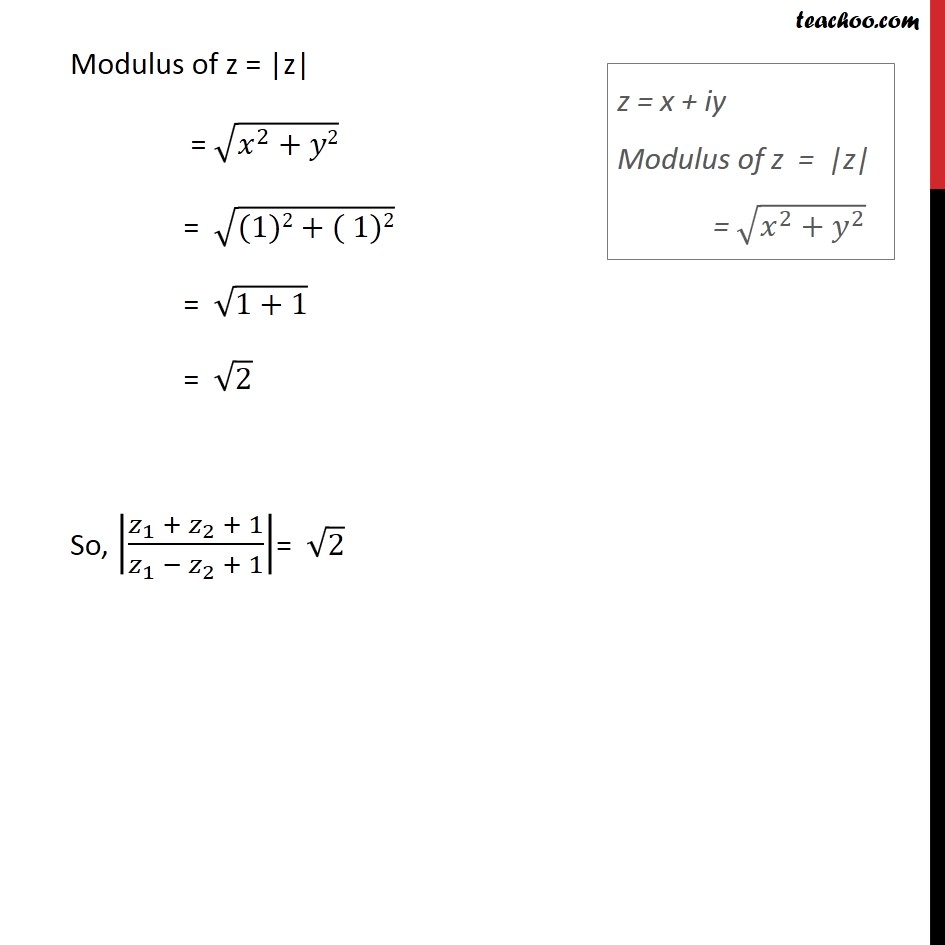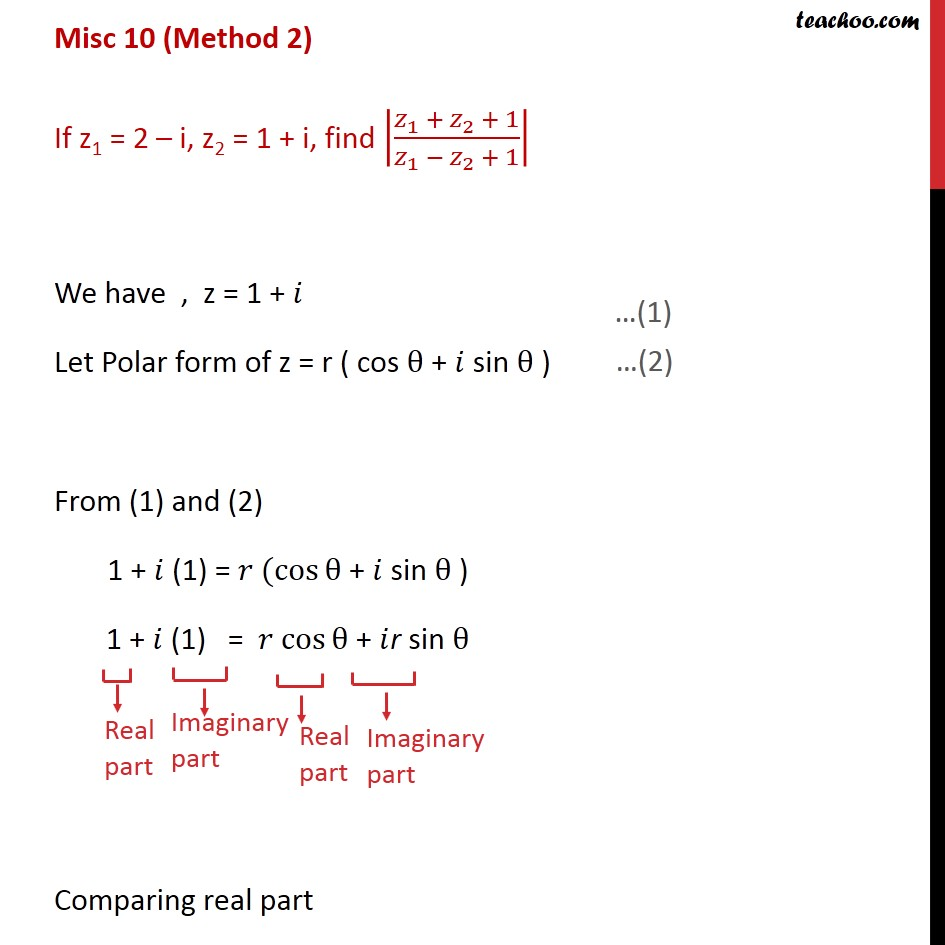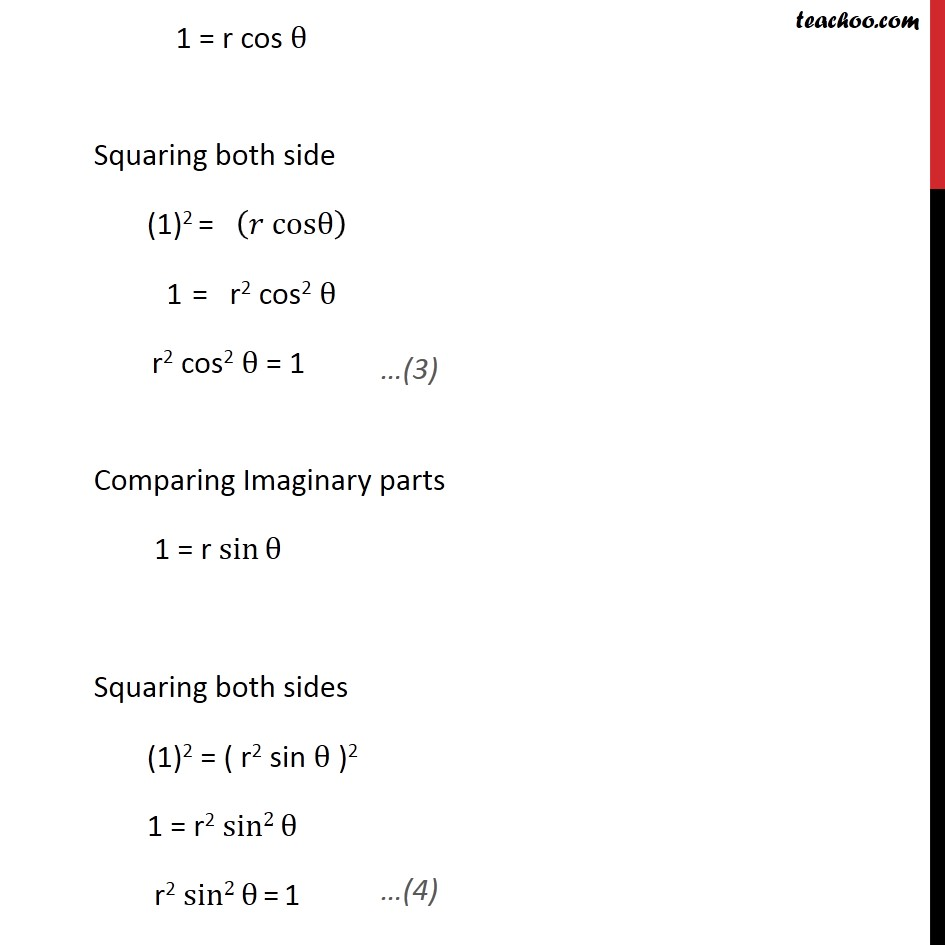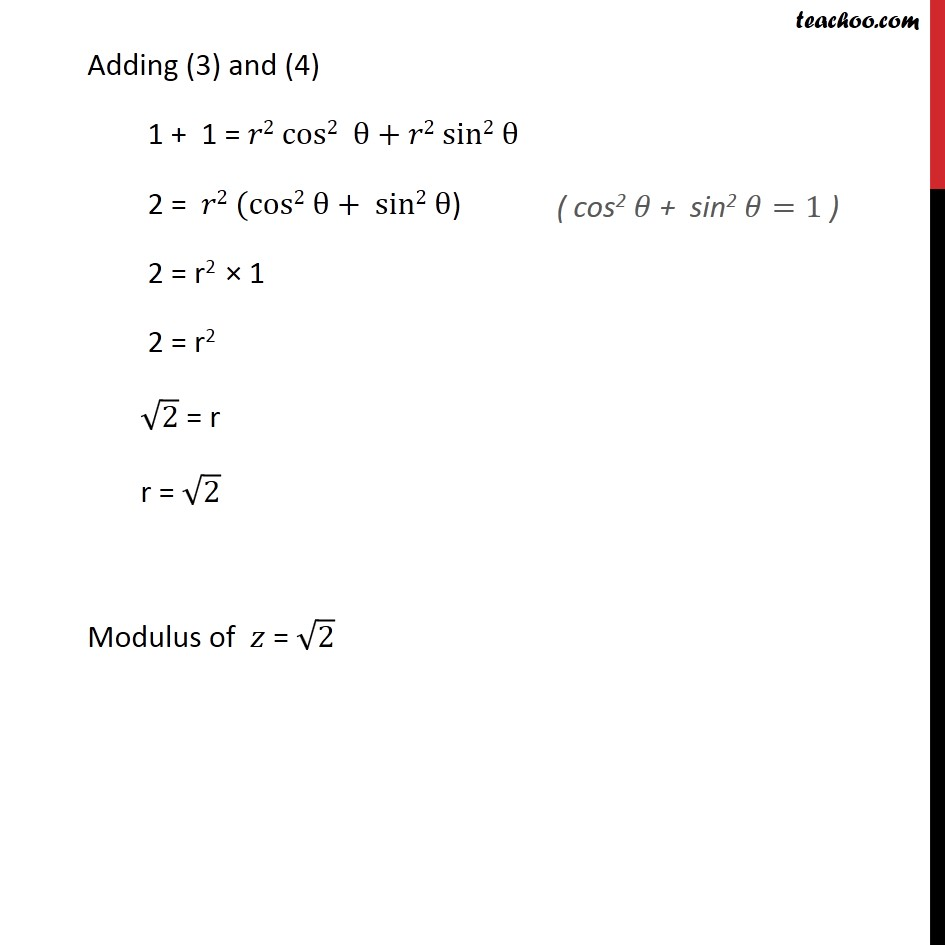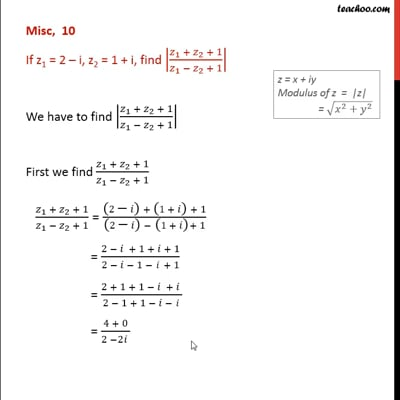This video is only available for Teachoo black users

### Transcript

Misc 10 (Method 1) If z1 = 2 – i, z2 = 1 + i, find |(𝑧_1 + 𝑧_2 + 1)/(𝑧_1 − 𝑧_2 + 1)| We have to find |(𝑧_1 + 𝑧_2 + 1)/(𝑧_1 − 𝑧_2 + 1)| First we find (𝑧_1 + 𝑧_2 + 1)/(𝑧_1 − 𝑧_2 + 1) (𝑧_1 + 𝑧_2 + 1)/(𝑧_1 − 𝑧_2 + 1) = ("(" 2"−" 𝑖")" + "(" 1+ 𝑖") " + 1)/("(" 2"−" 𝑖")" −" (" 1+ 𝑖")" + 1) = (2 − 𝑖 + 1 + 𝑖 + 1)/(2 − 𝑖 − 1 −" " 𝑖 + 1) = (2 + 1 + 1 − 𝑖 + 𝑖 )/(2 − 1 + 1 − 𝑖 −" " 𝑖) = (4 + 0)/(2 −2𝑖 ) = 4/(2 (1 − 𝑖) ) = 4/(2 (1 − 𝑖) ) = 2/((1 − 𝑖) ) Rationalizing = 2/(1 − 𝑖) × (1 + 𝑖)/(1 + 𝑖) = (2 (1 + 𝑖))/((1 − 𝑖) (1 + 𝑖)) Using (a – b) (a + b) = a2 - b2 = (2(1 + 𝑖))/((1)2 − (𝑖)2) Putting i2 = −1 = (2 (1 + 𝑖 ))/(1 −(−1) ) = (2(1 + 𝑖))/(1 + 1) = (2 (1 + 𝑖))/2 = 1 + 𝑖 Hence, (𝑧_1 + 𝑧_2 + 1)/(𝑧_1 − 𝑧_2 + 1) = 1 + 𝑖 Now we find |(𝑧_1 + 𝑧_2 + 1)/(𝑧_1 − 𝑧_2 + 1)| i.e. |1 + 𝑖| Complex number z is of the form 𝑥 + 𝑖 𝑦 Here x = 1 and y = 1 Modulus of z = |z| = √(𝑥^2+𝑦2) = √((1)2+( 1)2) = √(1+1) = √2 So, |(𝑧_1 + 𝑧_2 + 1)/(𝑧_1 − 𝑧_2 + 1)|= √2 Misc 10 (Method 2) If z1 = 2 – i, z2 = 1 + i, find |(𝑧_1 + 𝑧_2 + 1)/(𝑧_1 − 𝑧_2 + 1)| We have , z = 1 + 𝑖 Let Polar form of z = r ( cos θ + 𝑖 sin θ ) From (1) and (2) 1 + 𝑖 (1) = 𝑟 (cos⁡θ + 𝑖 sin θ ) 1 + 𝑖 (1) = 𝑟 cos⁡θ + 𝑖r sin θ Comparing real part 1 = r cos θ Squaring both side (1)2 = (𝑟 cosθ) 1 = r2 cos2 θ r2 cos2 θ = 1 Comparing Imaginary parts 1 = r〖 sin〗⁡θ Squaring both sides (1)2 = ( r2 sin θ )2 1 = r2 sin2⁡θ r2 sin2⁡θ = 1 Adding (3) and (4) 1 + 1 = 𝑟2 cos2 θ + 𝑟2 sin2 θ 2 = 𝑟2 (cos2 θ + sin2 θ) 2 = r2 × 1 2 = r2 √2 = r r = √2 Modulus of 𝑧 = √2